# 4. Analyzing the Feature Space for Further Improvement of Search Efficiency

## 4. Analyzing the Feature Space for Further Improvement of Search Efficiency

### 4.1 Distinctiveness of Nearest Neighbors in Feature Space

###### Indistinctive Nearest Neighbors

When we use the nearest-neighbor search (NN search), we expect that found neighbors are much closer than the others. However, this intuition is sometimes incorrect in high dimensional space. For example, when points are uniformly distributed in a unit hypercube, the distance between two points is almost the same for any combination of two points. Figure 27.10 shows the minimum, the average, and the maximum of the distances among 100,000 points generated at random in a unit hypercube. As shown in the figure, the minimum of the distances grows drastically as the dimensionality increases and the ratio of the minimum to the maximum increases up to 24 % in 16 dimensions, 40 % in 32 dimensions, and 53 % in 64 dimensions. Thus, the distance to the nearest neighbor is 53 % or more of the distance to the farthest point in 64-dimensional space. In this case, we can consider the nearest neighbor to be indistinctive, because the difference between the nearest neighbor and the others is negligible, i.e., the other points are as close to the query point as the nearest neighbor is. From the perspective of the similarity retrieval, when the nearest neighbor is indistinctive, the nearest neighbor has almost the same similarity with the others and does not have distinctive similarity to the query.Figure 27.10: Distances among 100,000 points generated at random in a unit hypercube.

As Figure 27.10 shows, indistinctive nearest neighbors are more likely to occur as dimensionality increases. This characteristic can be verified by estimating the distance to k-th nearest neighbor. When N points are distributed uniformly within the hypersphere whose center is the query point, the expected distance to k-th nearest neighbor dkNN is obtained as follows :

 (27.2)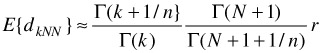where n is the dimensionality of the space and r is the radius of the hypersphere. Then, the ratio of the (k+1)-NN distance to the k-NN distance is obtained as follows :

 (27.3)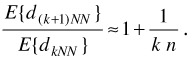Thus, when points are distributed uniformly around the query point, it is expected that the difference between the k-th and (k+1)-th nearest neighbors decreases as the dimensionality increases. This implies that indistinctive nearest neighbors are more likely to occur in high dimensional space than in low dimensional space.

Equation (27.3) also indicates that the ratio of d(k+1)NN to dkNN decreases monotonically as k increases. Therefore, the maximum of the ratio between two nearest neighbors is obtained for the first and the second nearest neighbors. From Equation (27.3), we can estimate the relative difference between the first and the second nearest neighbors when the dimensionality is n:

 (27.4)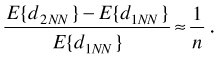This equation shows that the relative difference between the first and the second nearest neighbors decreases monotonically as the dimensionality increases (Figure 27.11). Only 20 % difference is expected at 5 dimensions, and only 10 % at 10 dimensions. This equation clearly shows that we could not expect strong distinctiveness of nearest neighbors for high dimensional uniform distribution.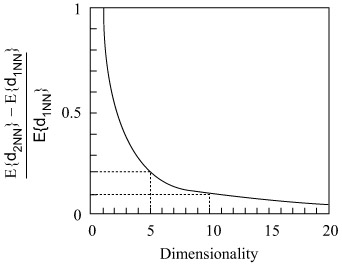Figure 27.11: Relative difference between the first and the second nearest neighbors.

###### Intrinsic Dimensionality of Feature Space

As shown above, indistinctive nearest neighbors are more likely to appear in high dimensional space, and the expected relative difference between the first and the second nearest neighbors is inversely proportional to the dimensionality of the distribution. However, this does not mean that high dimensional feature space is useless. We should note that the discussion above is based on the uniform distribution. If the data distribution over the entire feature space is uniform, we can say that the feature space is useless, but in real applications, the data distribution is not uniform at all.

Instead of assuming the uniform distribution to the entire space, we should employ the intrinsic dimensionality (or effective dimensionality) which is determined by local characteristics of the data distribution . For example, when the data distribution is governed by a number of dominant dimensions, the intrinsic dimensionality is given by the number of such dominant dimensions. In addition, the intrinsic dimensionality may not be consistent over the data set but vary from one local region to another.

In real applications, we can expect that the data distribution is so skewed that the intrinsic dimensionality could be much smaller than the dimensionality of the feature space. Therefore, we might have indistinctive nearest neighbors in one region but could have distinctive ones in another. In a region with low intrinsic dimensionality, we can expect distinctive nearest neighbors, while we cannot expect distinctive ones in a region with high intrinsic dimensionality. Thus, the intrinsic dimensionality is the important clue for estimating the distinctiveness of the nearest neighbor.

###### Harmful effect of indistinctive NNs

Indistinctive nearest neighbors have a harmful effect on the similarity retrieval with the following respects:

1. NN search performance is degraded.

When the nearest neighbor is indistinctive, there exist many points that have almost the same similarity with the nearest neighbor. Since these points are very strong candidates for the nearest neighbor, NN search operation is forced to examine many points before determining the true nearest neighbor. This degrades the performance of NN search operation.

2. Less informative result is returned.

When the nearest neighbor is indistinctive, NN search operation returns the closest point among many strong candidates that have almost the same similarity with the nearest neighbor. This means that all of the candidates have slight differences with each other. It is not informative for users to choose the nearest neighbor from plenty of similar candidates.

These effects are extremely harmful to the retrieval systems with human-computer interaction. When the nearest neighbor is indistinctive, the system forces users to wait until a less informative result is answered with slow response. Thus, it is necessary to handle indistinctive nearest neighbors appropriately in order to achieve efficient similarity retrieval of multimedia information.

### 4.2 Estimating the Distinctiveness of Nearest Neighbors

###### Determination of indistinctive NNs

As mentioned above, the intrinsic dimensionality plays an important role in determining the distinctiveness of the nearest neighbor. Therefore, we devised a probabilistic method which determines the distinctiveness of the nearest neighbor based on the intrinsic dimensionality. In the first place, we coined a definition of indistinctive nearest neighbors as follows:

Definition 1: Let dNN be the distance from the query point to a nearest neighbor. Then, the nearest neighbor is indistinctive if more than or equal to Nc points exist within the range of dNN to Rp dNN from the query point.

Here, Rp (>1) and Nc (>1) are controllable parameters (Figure 27.12). Rp determines the proximity of the query point and Nc determines the congestion of the proximity. For example, we set 1.84 to Rp and 48 to Nc at the experiment described in the following section.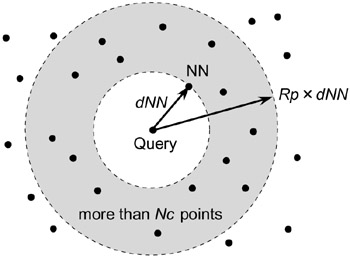Figure 27.12: Definition of the indistinctive NN.

As dimensionality increases, we are more likely to have plenty of points in the similar distance with the nearest neighbor. This causes congestion in the proximity of the query point. The above definition determines indistinctive nearest neighbors by detecting the congestion in the proximity. This characteristic can be clearly stated by estimating the probability of congestion. We call this probability the rejection probability. When points are distributed uniformly in a local region with the intrinsic dimensionality n, the probability that Nc points exist in the proximity specified by Rp is obtained as follows:

 (27.5)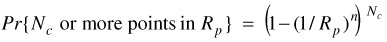According to the definition above, the nearest neighbor is indistinctive when Nc points exist in the proximity specified by Rp. Therefore, Equation (27.5) corresponds to the probability that the nearest neighbor is regarded as being indistinctive when the intrinsic dimensionality is n. This probability increases monotonically as the intrinsic dimensionality increases. Figure 27.13 shows the rejection probability when Rp is 1.84471 and Nc is 48.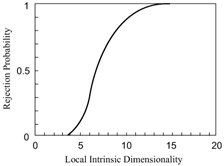Figure 27.13: Rejection probability when Rp is 1.84471 and Nc is 48.

###### Distinctiveness-Sensitive NN Search

In order to circumvent the harmful effect of indistinctive nearest neighbors, we developed a new NN search algorithm for multidimensional index structures with applying the distinctiveness estimation method described above . The algorithm tests the distinctiveness of the nearest neighbor in the course of search operation. Then, when it finds the nearest neighbor to be indistinctive, it quits the search and returns a partial result. We call this NN search the Distinctiveness-Sensitive Nearest-Neighbor Search since it is sensitive to the distinctiveness of the nearest neighbor. This algorithm not only enables us to determine the distinctiveness of the nearest neighbor but also enables us to cut down the search cost as shown in the following section.

The distinctiveness-sensitive NN search algorithm is an extension of the basic NN search algorithm. The main idea of the extension is counting the number of points that are located within the proximity of the query point in the course of search operation. When it finds the nearest neighbor to be indistinctive, it quits the search and returns the partial result as shown in Figure 27.14. When j-th nearest neighbor is found to be indistinctive during k-nearest neighbor search, the search is terminated and candidates on the termination are returned for j-th to k-th nearest neighbors. Thus, the mixture of the exact nearest neighbors and the nearest neighbor candidates is returned when the indistinctive nearest neighbor is found during the search operation. This algorithm not only enables us to determine the distinctiveness of nearest neighbors but also enables us to cut down the search cost since this algorithm avoids pursuing exact answers for indistinctive nearest neighbors.Figure 27.14: Search result of the distinctiveness-sensitive NN search.

### 4.3 Experimental Evaluation

We evaluated the performance of the distinctiveness-sensitive NN search with applying it to the similarity retrieval of images. The data set is 60,195 images of Corel Photo Collection contained in the product called Corel Gallery 1,000,000. The similarity of images is measured in terms of the color histogram. Munsell color system is used for the color space. It is divided into nine subspaces: black, gray, white, and six colors. For each image, histograms of four sub-regions, i.e., upper-left, upper-right, lower-left, and lower-right, are calculated in order to take account of the composition of the image. Four histograms are concatenated to compose a 36-dimensional feature vector. Similarity of images is measured by Euclidean distance among these 36-dimensional vectors. We measured the performance of finding 100 nearest neighbors with employing every image as a query. Therefore, one of the found nearest neighbors is the query image itself. Experiments are conducted on Sun Microsystems Ultra 60 (CPU: UltraSPARC-11 360MHz, main memory: 512 Mbytes, OS: Solaris 2.6). Programs are implemented in C++. The VAMSplit R-tree  is employed as the index structure, since it has an efficient static construction algorithm suitable for the NN search in high dimensional space. The parameters Rp and Nc are set to 1.84471 and 48 respectively.

We compared the cost of the distinctiveness-sensitive NN search with that of the basic NN search. As shown in Figure 27.15, both the CPU time and the number of disk reads of the distinctiveness-sensitive NN search are remarkably reduced compared with those of the basic NN search. The CPU time is reduced by 75 % and the number of disk reads is reduced by 72 %. This result demonstrates that the distinctiveness-sensitivity NN search enables us to cut down the search cost. Although the reduction rate depends on the data set, this cost reduction capability of the distinctiveness-sensitive NN search should be advantageous to the interactive similarity retrieval systems that need quick response to users.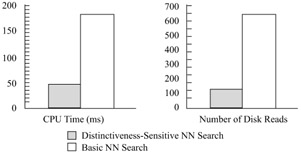Figure 27.15: Performance of the distinctiveness-sensitive NN search.

Table 27.1 shows the number of distinctive nearest neighbors found by the distinctiveness-sensitive NN search. Because we employed every image as a query, the total of the occurrence is equal to the number of images in the data set. Table 27.2 shows what kind of images are retrieved as distinctive nearest neighbors. We examined such search results that have relatively many distinctive nearest neighbors and then determined by hand what kind of images are retrieved as distinctive nearest neighbors. Figure 27.16 shows examples of search results when the number of distinctive nearest neighbors is relatively large. Due to the limitation of space, top 5 images are shown. The numerical value under each image is the distance from the query to the image. We can see that similar images are successfully retrieved by the distinctiveness-sensitive NN search.

Table 27.1: Number of distinctive NNs.

# of Distinctive NNs

# of Occurrence

100

0

(0%)

80 - 99

46

(0.08%)

60 - 79

1

(0.001%)

40 - 59

66

(0.1%)

20 - 39

124

(0.2%)

2 - 19

13918

(23.1%)

1

46040

(76.5%)

Total

60195

(100%)

Table 27.2: Categories of the results.

Category (Observed by Hand)

# of Distinctive NNs (at most)

Texture

92

Sky/ Sea

62

Portrait

35

Card

23

Firework

23

Sunset

19

Kung-Fu

18

Steamship

17

Desert

16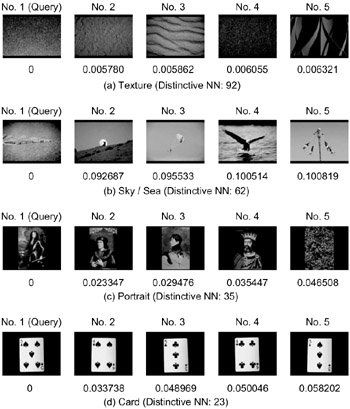Figure 27.16: Examples of search results.

The amazing result of Table 27.1 is that we obtained only one distinctive nearest neighbor for 46,040 images. Since each query image is chosen from the images in the data set, the obtained distinctive nearest neighbor is the query itself.

Therefore, we obtained no distinctive nearest neighbor except for the query image. In this case, the query image is surrounded by plenty of neighbors that have almost the same similarity to the query. Since Corel Photo Collection collects a wide variety of photos, it is not strange that an image has no similar one in the collection. In addition, the collection contains some texture photos. In this case, we have plenty of images with small difference. Figure 27.17 shows examples of search results when we obtained no distinctive nearest neighbors except for the query image. Due to the space limitation, top 5 images are shown. Figure 27.17 (a) is the case that the query image is surrounded by plenty of dissimilar images, while Figure 27.17 (b) is the case that the query image is surrounded by plenty of images with small difference. These examples illustrate that the distinctiveness-sensitive NN search allows us to see how retrieved images are significantly close to the query image. This capability should be advantageous to the interactive information retrieval systems.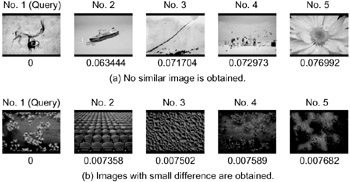Figure 27.17: Examples of results when no distinctive NN is found except for the query.

In order to validate the results of the distinctiveness-sensitive NN search, we plotted the distribution of nearest neighbors in two-dimensional form. Figure 27.18 shows the examples of the distribution of nearest neighbors; (a) is the case that no distinctive nearest neighbor is found (Figure 27.17 (a)), while (b) is the case that 62 distinctive nearest neighbors are found (Figure 27.16 (b)). The query image is located at the center. The images of 99 nearest neighbors are placed so that the distance from the query image to each image in the figure is proportional to the distance between their feature vectors in the feature space. The inner dashed circle indicates the distance to the 1st nearest neighbor, while the outer solid circle the 99th nearest neighbor. Figure 27.18(a) and (b) are scaled so that their outer solid circles are equal in size. The orientation of each nearest neighbor is not important in this illustration; it is determined by the first and second principal components of the feature vectors of nearest neighbors. As the figure shows, the distribution of nearest neighbors differs significantly from one query point to another. The broader the distribution is, the more the distinctive nearest neighbors are obtained. This tendency meets the aim of the distinctiveness-sensitive NN search, i.e., detecting the congestion of nearest neighbors.Figure 27.18: Examples of the distribution of 99 nearest neighbors.

In this experiment, we used still photo images as an example of multimedia information but the similar characteristics of the feature space, i.e., the skewness of distribution and the variety of the distinctiveness of nearest neighbors, can be observed in other types of multimedia information, e.g., video frames, face images, object appearances, etc.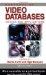Handbook of Video Databases: Design and Applications (Internet and Communications)
ISBN: 084937006X
EAN: 2147483647
Year: 2003
Pages: 393